You are currently offline. Some features of the site may not work correctly.

# Theory of equations

In algebra, the theory of equations is the analysis of the nature and algebraic solutions of algebraic equations (also called polynomial equations… Expand
Wikipedia

## Papers overview

Semantic Scholar uses AI to extract papers important to this topic.
2015
2015
• ICALP
• 2015
• Corpus ID: 586046
We show that, given an equation over a finitely generated free group, the set of all solutions in reduced words forms an… Expand
•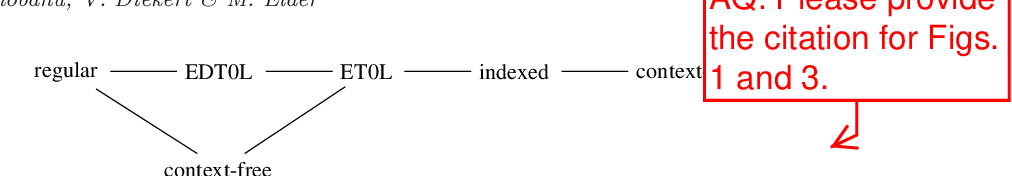•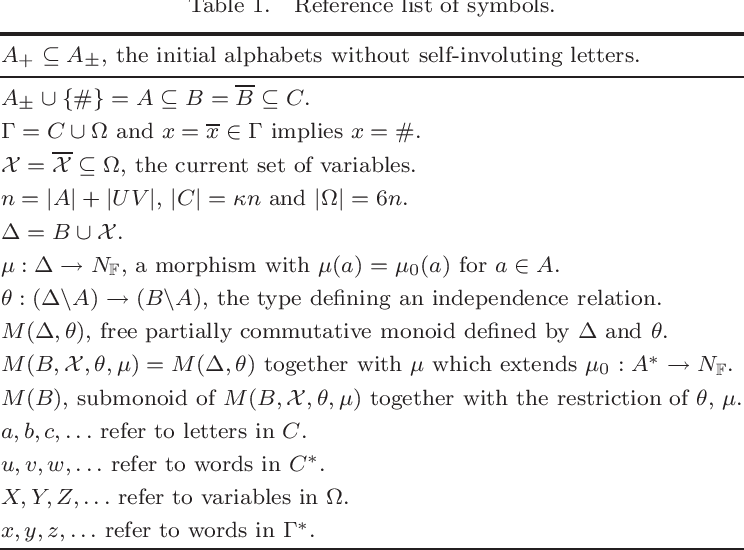•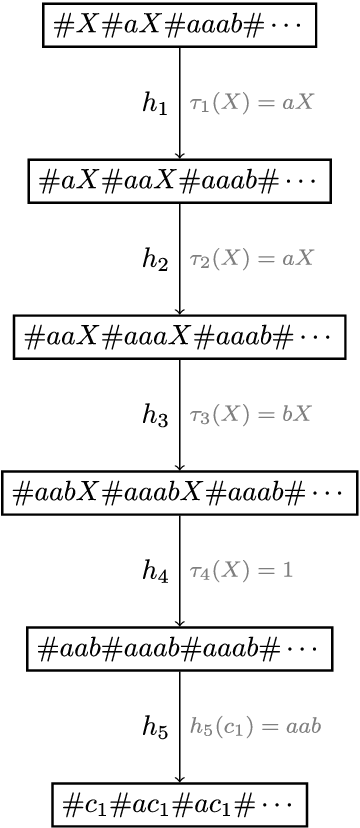Is this relevant?
2007
2007
T H E first part of this paper will treat of Galois' relations to Lagrange, the second part will sketch the manner in which… Expand
Is this relevant?
2005
2005
The paper deals with the problem of integration of equations of motion in nonholonomic systems. By means of well-known theory of… Expand
Is this relevant?
2005
2005
• Inf. Comput.
• 2005
• Corpus ID: 121231844
It is well-known that the existential theory of equations in free groups is decidable. This is a celebrated result of Makanin… Expand
Is this relevant?
2003
2003
• Theory of Computing Systems
• 2003
• Corpus ID: 22979729
Abstract We prove that the existential theory of equations with normalized rational constraints in a fixed graph product of… Expand
•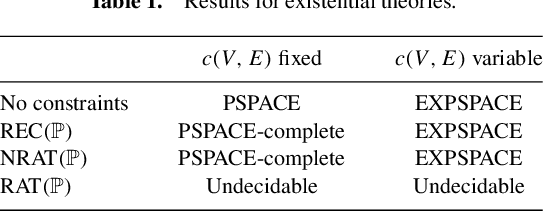Is this relevant?
2002
2002
• STACS
• 2002
• Corpus ID: 13121607
We prove that the existential theory of equations with normalized rational constraints in a fixed graph product of finite monoids… Expand
Is this relevant?
Highly Cited
1994
Highly Cited
1994
These lecture notes are devoted to the theory of “equations of associativity” describing geometry of moduli spaces of 2D… Expand
•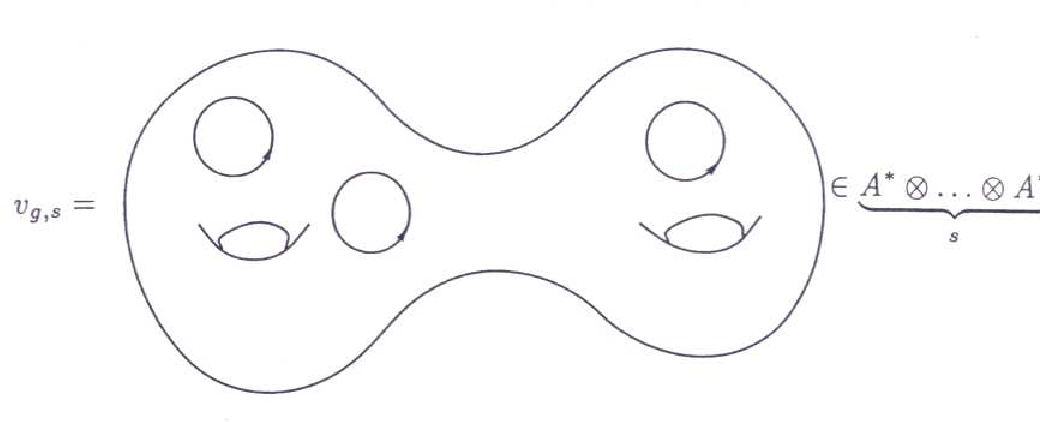•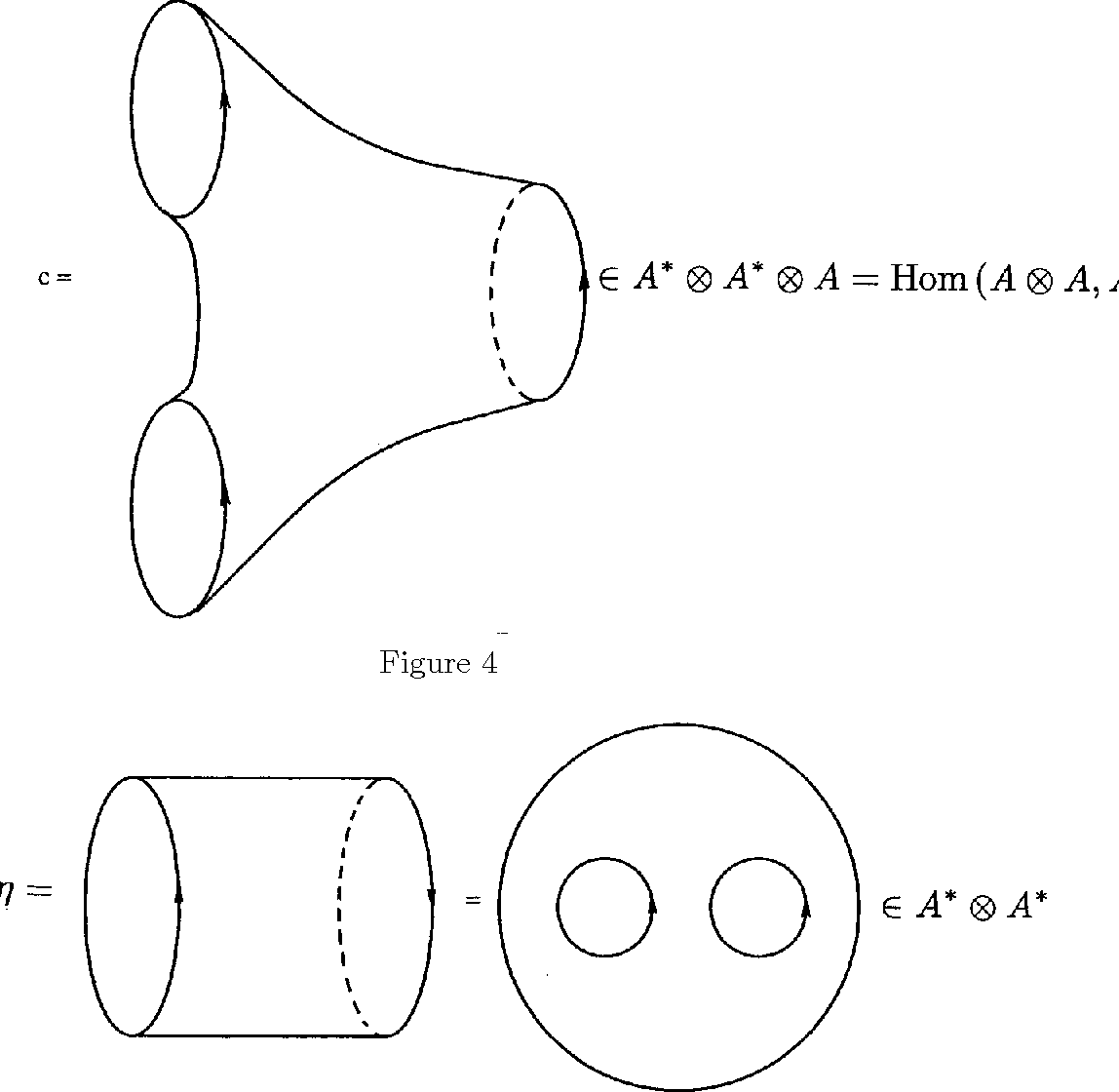•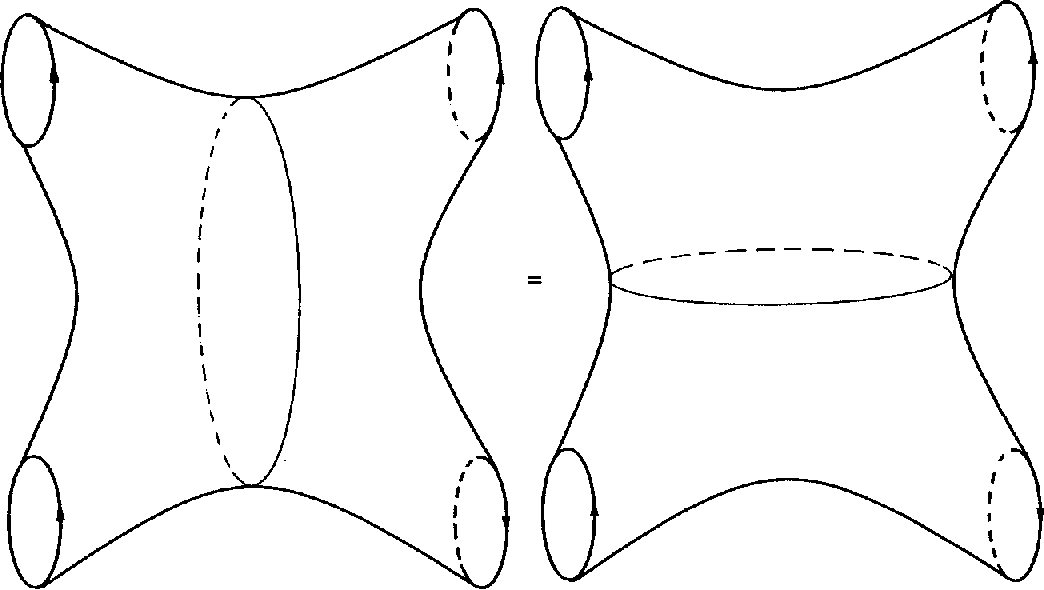•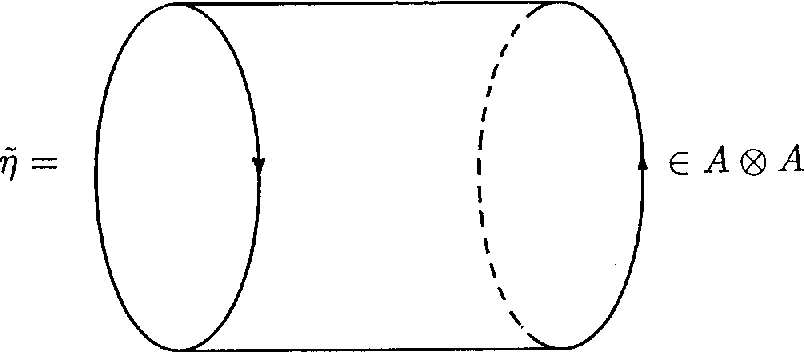•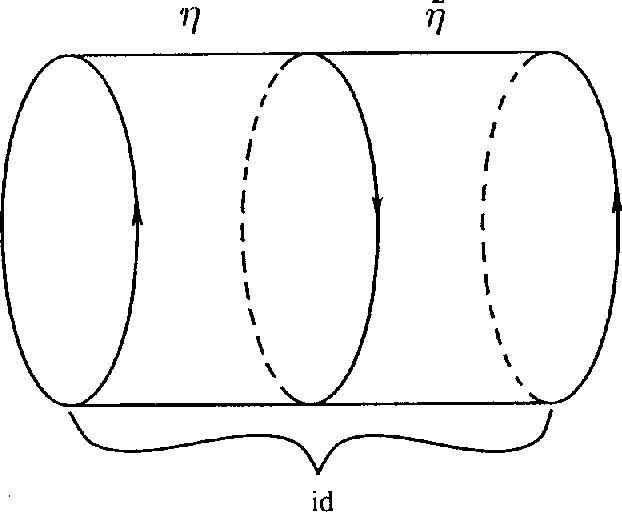Is this relevant?
1952
1952
If f(x) represents the function a1x n + a2x n −1 + … + an + 1 and f(a) denotes the value of the function when x is replaced by… Expand
Is this relevant?
1948
1948
THE contents of this volume fall into three clearly marked sections. Chapters 1–4 (pp. 1–105) lead up to the location and… Expand
Is this relevant?
1940
1940
THIS book is founded upon the author's earlier work but is written “in a more expansive style with many illustrations either… Expand
Is this relevant?